# Feature column a tutorial

Posted by chunyang on September 11, 2020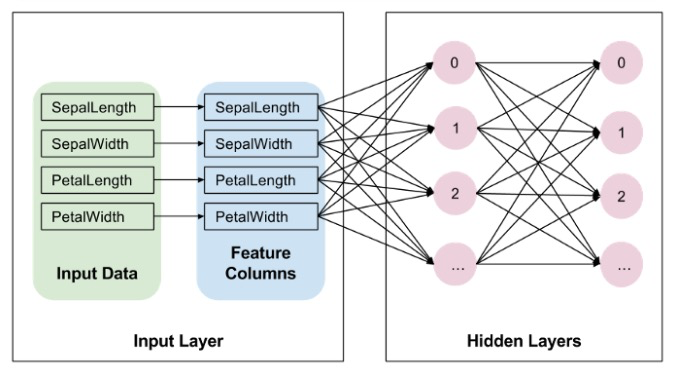• 什么是 Tensorflow 中的 feature column
• 数值的 feature column
• 分桶 feature column
• 分类 feature column
• 指示 feature column
• 向量化 feature column
• 哈希的 feature column
• 交叉的 feature column
• 在 tf.keras.models 中如何使用
• 在 estimator 中如何使用（线性和基于树的模型）

### 特征列 feature column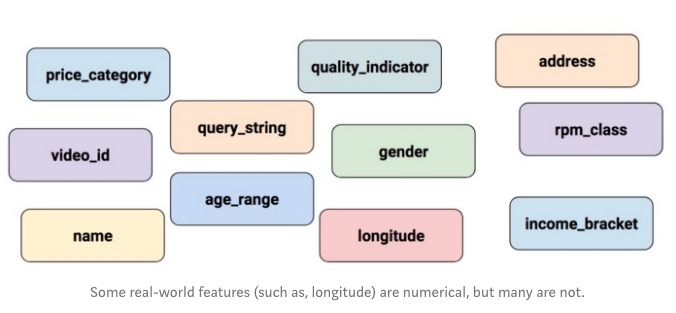### 深度神经网络输入

• kitchenware
• electronics
• sports

• 0: kitchenware 不存在
• 0: electronics 不存在
• 1: sports 存在

### Feature columns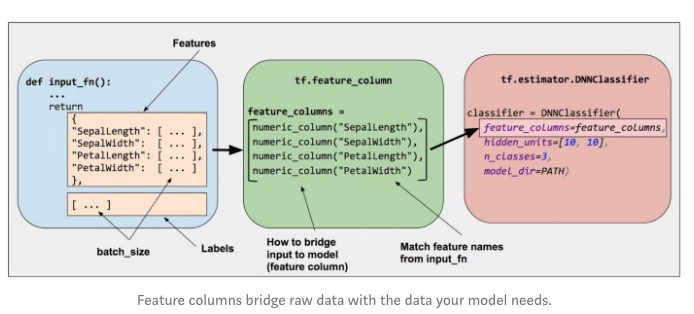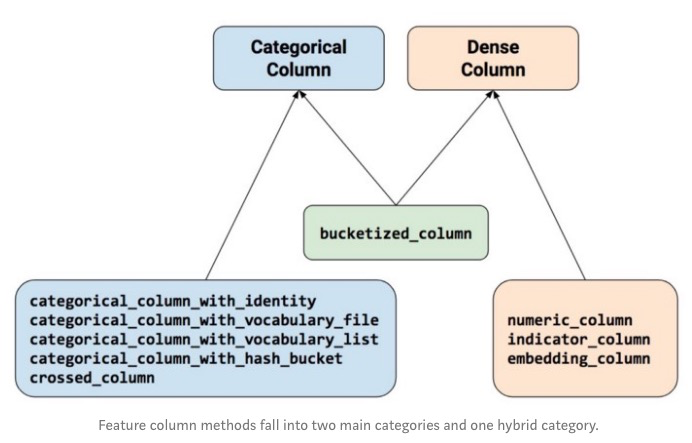### 引入 Tensorflow 和其它库

from __future__ import absolute_import, division, print_function, unicode_literals
import numpy as np
import pandas as pd
import tensorflow as tf
from tensorflow import feature_column
from tensorflow.keras import layers


### 构造示例数据

data = {'marks': [55,21,63,88,74,54,95,41,84,52],
'average','good','average','good','average'
],
'point': ['c','f','c+','b+','b','c','a','d+','b+','c']}


### 示例数据

df = pd.DataFrame(data)
df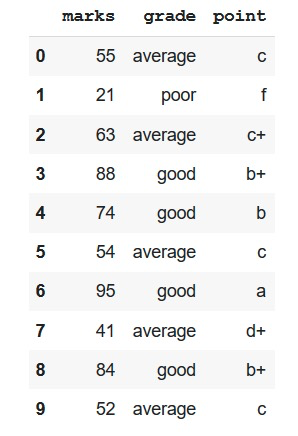### 演示几种不同类型的特征列

# A utility method to show transromation from feature column
def demo(feature_column):
feature_layer = layers.DenseFeatures(feature_column)
print(feature_layer(data).numpy())


### 数值列 (Numeric columns)

marks = feature_column.numeric_column("marks")
demo(marks)
>> [[55.]
[21.]
[63.]
[88.]
[74.]
[54.]
[95.]
[41.]
[84.]
[52.]]


### 分桶列 (Bucketized columns)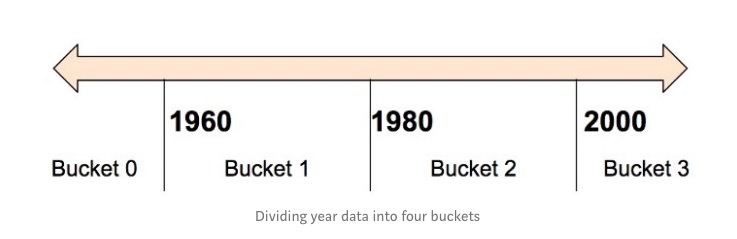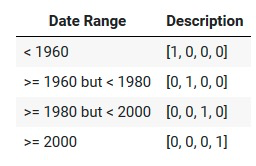marks_buckets = feature_column.bucketized_column(
marks, boundaries=[30,40,50,60,70,80,90]
)
demo(marks_buckets)
>> [[0. 0. 0. 1. 0. 0. 0. 0.]
[1. 0. 0. 0. 0. 0. 0. 0.]
[0. 0. 0. 0. 1. 0. 0. 0.]
[0. 0. 0. 0. 0. 0. 1. 0.]
[0. 0. 0. 0. 0. 1. 0. 0.]
[0. 0. 0. 1. 0. 0. 0. 0.]
[0. 0. 0. 0. 0. 0. 0. 1.]
[0. 0. 1. 0. 0. 0. 0. 0.]
[0. 0. 0. 0. 0. 0. 1. 0.]
[0. 0. 0. 1. 0. 0. 0. 0.]]


### 分类特征 (Categorical columns)

#### 指示列 (Indicator columns)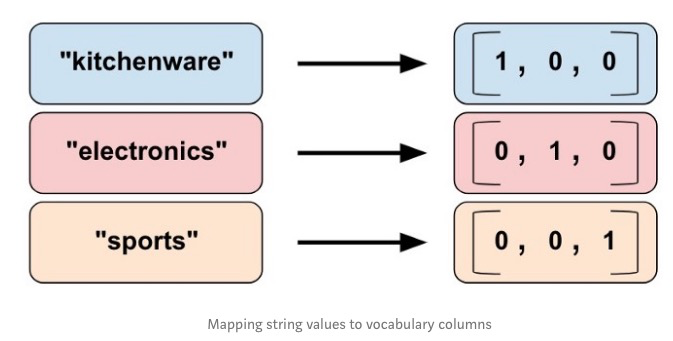grade = feature_column.categorical_column_with_vocabulary_list(
>> [[0. 1. 0.]
[1. 0. 0.]
[0. 1. 0.]
[0. 0. 1.]
[0. 0. 1.]
[0. 1. 0.]
[0. 0. 1.]
[0. 1. 0.]
[0. 0. 1.]
[0. 1. 0.]]


#### Point 列编码为指示列（Point column as indicator_column)

point = feature_column.categorical_column_with_vocabulary_list(
‘point’, df[‘point’].unique())
point_one_hot = feature_column.indicator_column(point)
demo(point_one_hot)
>> [[1. 0. 0. 0. 0. 0. 0.]
[0. 1. 0. 0. 0. 0. 0.]
[0. 0. 1. 0. 0. 0. 0.]
[0. 0. 0. 1. 0. 0. 0.]
[0. 0. 0. 0. 1. 0. 0.]
[1. 0. 0. 0. 0. 0. 0.]
[0. 0. 0. 0. 0. 1. 0.]
[0. 0. 0. 0. 0. 0. 1.]
[0. 0. 0. 1. 0. 0. 0.]
[1. 0. 0. 0. 0. 0. 0.]]


#### Point 列编码为向量列 （Point column as embedding_column)

# Notice the input to the embedding column is the categorical column
# we previously created
point_embedding = feature_column.embedding_column(point, dimension=4)
demo(point_embedding)
>>[[ 0.6905385  -0.08270663  0.15046535 -0.3439266 ]
[ 0.56737125  0.06695139 -0.58371276  0.49233127]
[ 0.4538004  -0.03839593 -0.4058998  -0.1113084 ]
[-0.50984156 -0.11315092  0.39700866  0.09811988]
[ 0.35654882  0.41658938 -0.67096025 -0.2758388 ]
[ 0.6905385  -0.08270663  0.15046535 -0.3439266 ]
[ 0.58311635  0.6259656  -0.27828178  0.14894487]
[-0.721768   -0.0898371   0.5906883  -0.4207294 ]
[-0.50984156 -0.11315092  0.39700866  0.09811988]
[ 0.6905385  -0.08270663  0.15046535 -0.3439266 ]]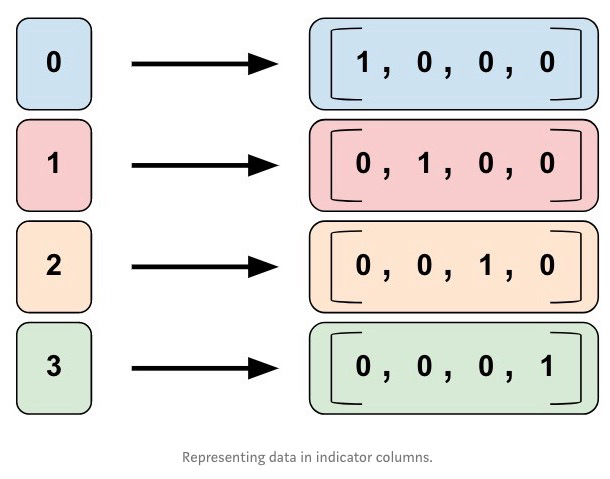• dog
• spoon
• scissors
• guitar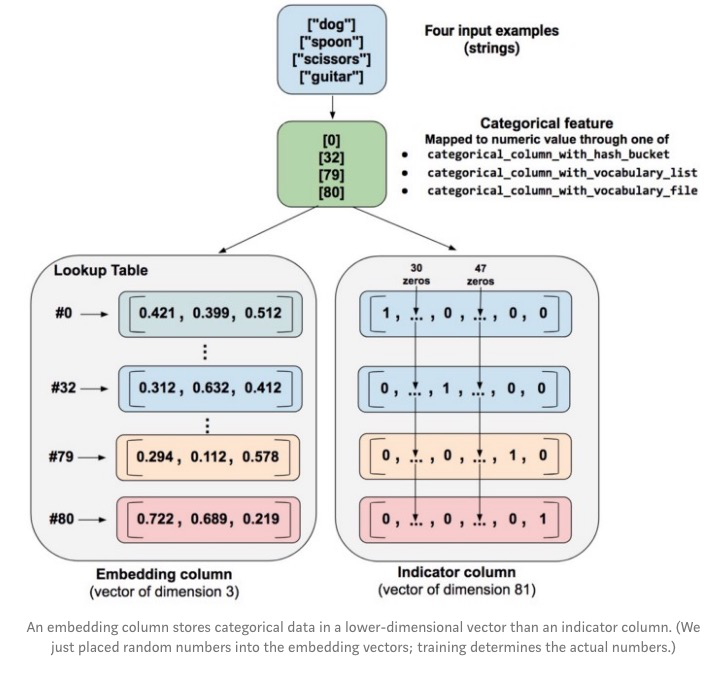• 指示列。函数将每一个数值分类值转化为一个 81 维的向量，分类值位置设置为 1，其它位置为 0.
• 向量列。函数将数值的分类值（0,32,79,80) 设置为一个查找表的索引。每一个查找的位置包含长度为 3 的向量

### 哈希特征列 (Hashed feature column)

point_hashed = feature_column.categorical_column_with_hash_bucket(
'point', hash_bucket_size=4
)
demo(feature_column.indicator_column(point_hashed))
>>
[[1. 0. 0. 0.]
[1. 0. 0. 0.]
[0. 1. 0. 0.]
[0. 0. 0. 1.]
[0. 0. 1. 0.]
[1. 0. 0. 0.]
[0. 0. 0. 1.]
[1. 0. 0. 0.]
[0. 0. 0. 1.]
[1. 0. 0. 0.]]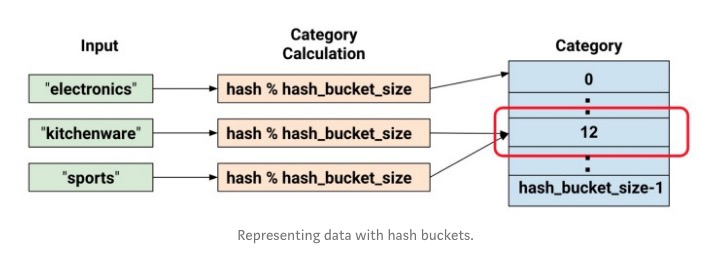### 交叉列 (Crossed feature columns)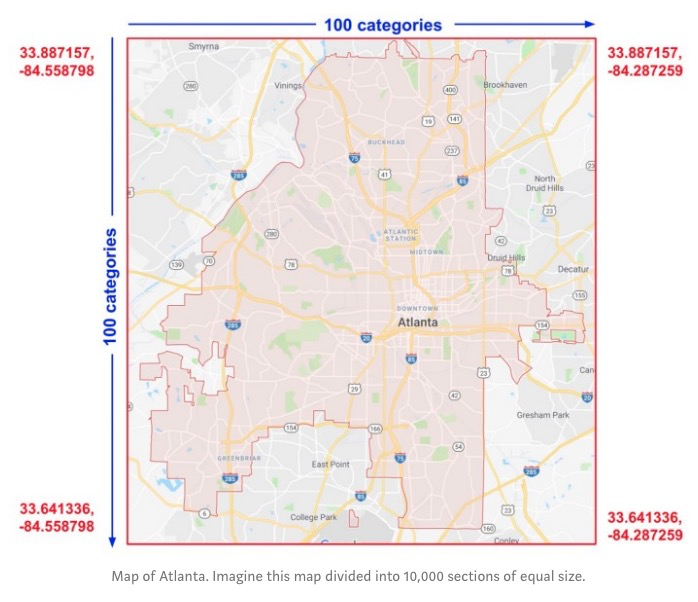crossed_feature = feature_column.crossed_column(
)
demo(feature_column.indicator_column(crossed_feature))
>>
[[0. 0. 0. 0. 0. 0. 0. 0. 1. 0.]
[0. 0. 0. 0. 1. 0. 0. 0. 0. 0.]
[0. 0. 0. 0. 1. 0. 0. 0. 0. 0.]
[0. 0. 0. 0. 1. 0. 0. 0. 0. 0.]
[1. 0. 0. 0. 0. 0. 0. 0. 0. 0.]
[0. 0. 0. 0. 0. 0. 0. 0. 1. 0.]
[0. 0. 0. 0. 0. 1. 0. 0. 0. 0.]
[0. 0. 0. 0. 0. 0. 1. 0. 0. 0.]
[0. 0. 0. 0. 1. 0. 0. 0. 0. 0.]
[0. 0. 0. 0. 0. 0. 0. 0. 1. 0.]]


• 特征名字： input_fn 返回的字典的键名字
• 任意一个分类类，除了 categorical_colun_with_hash_bucket （因为交叉列会将输入哈希，译注：重复哈希可能会有问题）

### 其它

#### 非 eager 代码

• 当使用哈希相关的功能时，会需要初始化表
import numpy as np
import pandas as pd
import tensorflow as tf
from tensorflow import feature_column
from tensorflow.keras import layers

data = {'marks': [55,21,63,88,74,54,95,41,84,52],
'average','poor','average','good','good','average',
'good','average','good','average'
],
'point': ['c','f','c+','b+','b','c','a','d+','b+','c']}

df = pd.DataFrame(data)

sess = tf.Session()

# A utility method to show transformation from feature column
def demo(feature_column):
feature_layer = layers.DenseFeatures(feature_column)
tensor = feature_layer(data)
sess.run(tf.global_variables_initializer())
sess.run(tf.tables_initializer())
print(sess.run(tensor))

marks = feature_column.numeric_column("marks")
demo(marks)

marks_buckets = feature_column.bucketized_column(
marks, boundaries=[30,40,50,60,70,80,90]
)
demo(marks_buckets)

point = feature_column.categorical_column_with_vocabulary_list(
'point', df['point'].unique())
point_one_hot = feature_column.indicator_column(point)
demo(point_one_hot)

point_embedding = feature_column.embedding_column(point, dimension=4)
demo(point_embedding)

point_hashed = feature_column.categorical_column_with_hash_bucket(
'point', hash_bucket_size=4)
demo(feature_column.indicator_column(point_hashed))

crossed_feature = feature_column.crossed_column(

categorical_column_with_identity 。这个是假设原始的特征就已经是数值化。后面可以继续使用这个值来进行编码为 indicator_column 或者 embedding_column 。